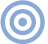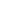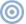# Features

## An Amplifier Listening Test Page 2

Our point about the proper use of chi-square is, by the way, quite well established statistically and not a matter of debate. The error made in this analysis is a very common one and is frequently singled out for discussion in statistical textbooks. For example, in his authoritative 1981 statistics text, Hays warns that "caution may be required in the application of chi-square tests to data where dependency among observations may be present, as is sometimes the case in repeated observations of the same individuals." Earlier, in his classic statistics text, McNemar (1962) pointed out that the assumption of independence is "violated when the total of the observed frequencies exceeds the total number of persons in the sample(s)." Such is the case with the data used in the Stereophile test. [See Sidebar—John Atkinson.]

The other error in the analysis of the Stereophile amplifier test derives from the unequal a priori presentation probabilities of "same" and "not same" trials. Mr. Hammond is correct in stating that if people were guessing randomly, this should not make a difference in the results. However, they were not guessing randomly, but rather had a very strong bias to say "not same." When these two deviations from 50/50 exist, predicted performance obtained by pure chance can differ greatly from 50%.

We will use some intuitive examples to explain how this works before we consider the amplifier test. First, let's assume that about 80% of the population is right-handed and 20% is left-handed. Suppose you were given the telephone book for a small city and asked to guess for each listing whether the person was right- or left-handed. How well would you do? If you guessed "left" and "right" equally often you would categorize exactly 50% of the people correctly: 40% of the people would be correctly named as right-handers (ie, half of the 80%, or 0.5 x 0.8), and another 10% of the people would be correctly named as left-handers (0.5 x 0.2).

If you were clever, you could do much better by categorizing them all as right-handers. That way you would be 80% correct: All of the right-handers would be correctly categorized for a score of 80%, and none of the left-handers would be correct, but since they make up 20% of the population, your accuracy would stand at 80%. To put it another way, without ever testing anyone for handedness you would still correctly categorize them 80% of the time. Clairvoyance? Of course not! With probabilities as skewed as this, 80% is exactly what is predicted.

Just for one more example, let's look at an in-between case: What would happen if you guessed 80% right-handers and 20% left-handers? This works out to be 68% "correct," not quite as good as the too-clever 80%, but much better than the mythical 50% "chance." Here is how the 68% is achieved: For the 80% of the population that is right-handed, you will guess that 80% are indeed right-handed and that 20% are left-handed, for an accuracy of 64%. For the left-handers, since you have no way of knowing who they are, you will also guess that 80% of them are right-handed. You will be wrong on them, but you will garner another 4% correct because you will guess that 20% of them are lefties, and 0.2 x 0.2 = 0.04. Hence, the total is 68%. Clearly, if we were testing to see if your ability to classify people by handedness was better than chance, we could use 50% as chance only if your guessing probability was 0.5 for left and 0.5 for right.

In the present case, we have to consider that 30 out of 56 (or 53.6%) presentations were different amplifiers and 26 of 56 were the same. If an observer guessed "different" on every trial without even listening, he or she would necessarily get a score of 53.6% correct. Given that they actually guessed "different" 63.1% of the time and "same" 36.9%, how would they do by chance? Let's figure it out. On the 53.6% of the trials in which the amplifiers were different, people would be assumed to guess that 63.1% were different, for an overall correct classification of 33.8% (0.536 x 0.631 = 0.338) of the total. On the 46.4% of trials where the two amplifiers were the same, people would be assumed to guess that 46.4% were "same" (46.4% = 100%-56.3%), and they would pick up another 17.1% (0.464 x 0.369), for a total of 50.9%.

So, if observers did not even listen to the amplifiers but simply guessed that 63.1% were different, they would get nearly 51% correct in this study. What we need to know is how much better they did by listening and whether the improvement is more than what chance would predict. People actually got 52.3% correct. They therefore did 1.4% better than chance expectation. Note that the reference point that corresponds to chance is 50.9%, not 50%.

To test whether the 1.4% improvement is statistically reliable, we need to use the chi-square test correctly. The unit of analysis is the individual (of which there are 505) rather than the response. Our test used the data in fig.2 of the Stereophile article, which shows the proportion of observers who got zero correct, one correct, two correct, and so on. We converted these to frequencies and compared them with the appropriate distribution with p=0.509 rather than 0.5. (This is like assuming that we are flipping a slightly unfair coin.) When all this is done, the chi-square is equal to 4.23. For a sample this size, the result indicates that this distribution of responses falls at about the 75% probability level. To translate the exact meaning of the test into plain English, the chi-square value indicates that strictly by chance we would expect to obtain a distribution at least as extreme as this one 75% of the time. In even plainer English, this means that the results don't mean anything at all!

For another way of looking at the results, consider just what a mean difference of 1.4% means in this case. It is consistent with the hypothesis that 1.4% of the population could judge correctly while the remaining 98.6% could not. In other words, all we need is about seven people out of the 505 to judge correctly for this mean difference to be generated. The remaining 498 might as well be deaf.ARTICLE CONTENTSX